Processing ......FreeComputerBooks.com Links to Free Computer, Mathematics, Technical Books all over the World

Analytic Combinatorics
🌠 Top Free Programming Books - 100% Free or Open Source!
• Title Analytic Combinatorics
• Author(s) Philippe Flajolet and Robert Sedgewick
• Publisher: Cambridge University Press; 1 edition (January 19, 2009)
• Hardcover: 824 pages
• eBook: PDF (826 pages, 11.8 MB)
• Language: English
• ISBN-10: 0521898064
• ISBN-13: 978-0521898065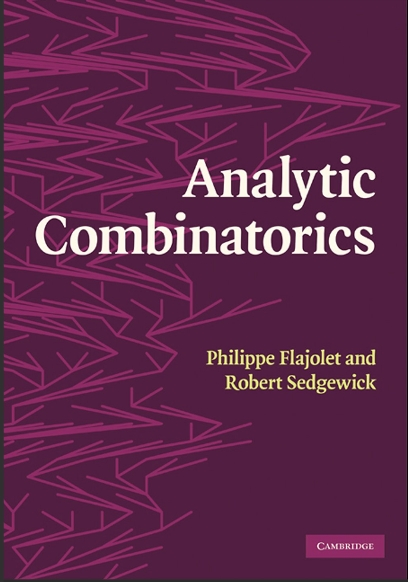Book Description

The definitive treatment of analytic combinatorics. This self-contained text covers the mathematics underlying the analysis of discrete structures, with thorough treatment of a large number of applications. Exercises, examples, appendices and notes aid understanding: ideal for individual self-study or for advanced undergraduate or graduate courses.

Reviews, Ratings, and Recommendations: Related Book Categories: Read and Download Links:Similar Books:
•Combinatorics Through Guided Discovery (Kenneth P. Bogart)

This book is an introduction to combinatorial mathematics, also known as combinatorics. The book focuses especially but not exclusively on the part of combinatorics that mathematicians refer to as 'counting'.

•Applied Combinatorics (Mitchel T. Keller, et al)

This is a text with more than enough material for a one-semester introduction to combinatorics. The original target audience was primarily computer science majors, but the topics included make it suitable for a variety of different students.

•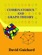An Introduction to Combinatorics and Graph Theory

This book walks the reader through the classic parts of Combinatorics and graph theory, while also discussing some recent progress in the area: on the one hand, providing material that will help students learn the basic techniques.

•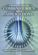Foundations of Combinatorics with Applications (Edward Bender)

This introduction to combinatorics, the foundation of the interaction between computer science and mathematics, is suitable for upper-level undergraduates and graduate students in engineering, science, and mathematics.

•Lists, Decisions and Graphs - With an Introduction to Probability

In this book, four basic areas of discrete mathematics are presented: Counting and Listing (Unit CL), Functions (Unit Fn), Decision Trees and Recursion (Unit DT), and Basic Concepts in Graph Theory (Unit GT).

•Magic Squares and Cubes (William Symes Andrews)

This book cover topics such as magic squares, magic cubes, the Franklin squares, magics and Pythagorean numbers, the theory of reversions, magic circles, spheres, and stars, and magic octahedroids, among other things.

•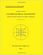Combinatorial Geometry with Application to Field Theory

Topics covered in this book include fundamental of combinatorics, algebraic combinatorics, topology with Smarandache geometry, combinatorial differential geometry, combinatorial Riemannian submanifolds, Lie multi-groups, etc.

•Notes on Convex sets, Polytopes, Polyhedra, Combinatorial Topology

This book may be viewed as a tutorial and a set of notes on convex sets, polytopes, polyhedra, combinatorial topology, Voronoi Diagrams and Delaunay Triangulations.

•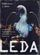LEDA: A Platform for Combinatorial and Geometric Computing

LEDA is a library of efficient data types and algorithms and a platform for combinatorial and geometric computing. This book, written by the main authors of LEDA, is the definitive account of how the system operates and how it can be used.

•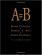A=B, by Marko Petkovsek, Herbert S. Wilf, et al

This book is of interest to mathematicians and computer scientists working in finite mathematics and combinatorics. It presents a breakthrough method for analyzing complex summations.

•Algebraic Combinatorics on Words (M. Lothaire)

This book is both a comprehensive introduction to the subject and a valuable reference source for researchers.

•Applied Combinatorics on Words (M. Lothaire)

The aim of this book is to present a unified treatment of some of the major fields of applications of combinatorics.

Book Categories
 :All CategoriesTop Free BooksRecent BooksMiscellaneous BooksComputer EngineeringComputer LanguagesComputer ScienceData Science/DatabasesJava and Java EE (J2EE)Linux and UnixMathematicsMicrosoft and .NETMobile ComputingNetworking and CommunicationsSoftware EngineeringSpecial TopicsWeb Programming
Other Categories